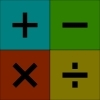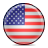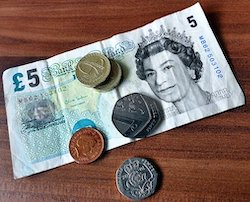#### You may also like### Worms

Place this "worm" on the 100 square and find the total of the four squares it covers. Keeping its head in the same place, what other totals can you make?### Which Scripts?

There are six numbers written in five different scripts. Can you sort out which is which?### Highest and Lowest

Put operations signs between the numbers 3 4 5 6 to make the highest possible number and lowest possible number.

# Would You Rather?

## Would You Rather?

If you are working in the USA units then go toWould you rather...Be given $60 \%$ of $2$ pizzas or $26 \%$ of $5$ pizzas?

Have $10 \%$ of $£5$ or $75 \%$ of $80$p?

Be bitten by $15 \%$ of $120$ mosquitoes or $8 \%$ of $250$ mosquitoes?Skip using a rope which is $54 \%$ of $105$cm long, or $88 \%$ of $2.75$m long?

Sit in a traffic jam for $33 \%$ of $2$ hours or $44 \%$ of $1$hr $40$mins?

Make sure you explain your reasons clearly.

Perhaps you'd like to make up some of your own questions like these and send them to us.

### Why do this problem?

This activity would be a good start to, or could follow on from, work on finding percentages of quantities. In whichever context it is used, it will provide a good assessment opportunity for you to find out what your pupils understand well and what they have difficulties with.

### Possible approach

These problems are probably best tackled as a group activity with children working in threes or fours. Give each group a set of these cards, five of which contain one each of the scenarios and one of which is blank. Challenge each group to decide which situation they would prefer and say that you will want them to explain how they came to their decisions.

Having given them a suitable length of time to work on the problems, bring everyone together. You could take a vote on each one to start with and then ask one group to explain how they came to that conclusion. Invite other groups to contribute different ways of working out the solution as well so that a range of methods are discussed.

You could then ask each group to make up their own question in a similar style which they could write on the blank card. These could then be swapped. Of course the group which poses the problem must have also worked out the solution!

### Key questions

How will you compare these two scenarios?
Can you express this percentage in any other way?
What do you estimate this percentage to be?

### Possible extension

Making up a similar problem (with its solution!), whose answer is not immediately obvious, will challenge many learners.

### Possible support

Calculators may be useful.